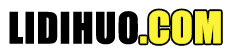# D3.js 选择 API

## 配置 API

```<script src = "https://d3js.org/d3-selection.v1.min.js"></script>
<script>
</script>
```

## 选择 API 方法

d3.selection() d3.select(selector) d3.selectAll(selector) selection.selectAll(selector) selection.filter(filter) selection.merge(other) d3.matcher(selector) d3.creator(name) selection.each(function) selection.call(function[, arguments…]) d3.local() local.set(node, value) local.get(node) local.remove(node)

### d3.select((selector))

```var body = d3.select("body");
```

```d3.select("p").style("color", "red");
```

### d3.selectAll((selector))

```var body = d3.selectAll("body");
```

```d3.selectAll("body").style("color", "red");
```

### selection.selectAll((selector))

```var b = d3.selectAll("p").selectAll("b");
```

### selection.filter(filter)

```var even = d3.selectAll("tr").filter(":nth-child(odd)");
```

### selection.merge(other)

```var rect = svg.selectAll("rect").data(data);
rect.enter().append("rect").merge(rect);
```

### d3.matcher((selector))

```var p = selection.filter(d3.matcher("p"));
```

### d3.creator(name)

```selection.append(d3.creator("p"));
```

### selection.each(function)

```parent.each(function(p, j) {
d3.select(this)
.selectAll(".child")
.text(function(d, i) { return "child " + d.name + " of " + p.name; });
});
```

### selection.call(function[, arguments…])

```function name(selection, first, last) {
selection.attr("first-name", first).attr("last-name", last);
}
```

```d3.selectAll("p").call(name, "Adam", "David");
```

### d3.local()

D3 local 允许您定义独立于数据的本地状态。

```var data = d3.local();
```

### local.set(node, value)

```selection.each(function(d)
{ data.set(this, d.value); });
local.get(node)
```